## 4.1.3 Continuity Equations

In order to obtain the continuity equations from the first Maxwell equation (4.1) the current density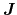has to be split in an electron and hole component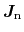and. Assuming the net concentration of ionized dopantsis time-invariant the following equation is obtained: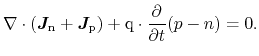(4.12)

(4.12) can be expressed in two different ways with the help of the quantity: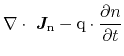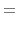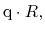(4.13)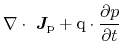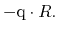(4.14)

This formulation givesthe meaning of the net generation or recombination of electrons and holes. As such, it can be modeled for the respective recombination/generation mechanisms, thereby (4.13) and (4.14) can be considered as two equations.

S. Vitanov: Simulation of High Electron Mobility Transistors